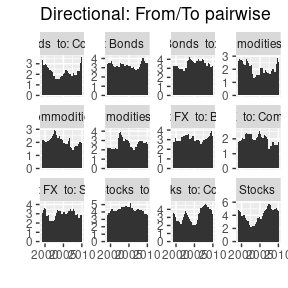# Introduction

This package provides useful and friendly functions to reproduce Diebold and Yilmaz (2012) papers. Its capabilities are not only for getting the directional indexes, but for plotting them too!

In this vignette we are showing how to get all results from Diebold and Yilmaz (2012).

### Making DY2012 reproducible

Let’s start loading the package and data. In order to confirm we have the correct data, let’s get the Table 1 from the paper.

options(repos = list(CRAN="http://cran.rstudio.com/"))
library(Spillover, quietly = TRUE)
data(dy2012)

Once we are sure we have the correct data, let’s get the spillover table, Table 2, this table is based on a VAR(4) and 10-day-ahead variance decomposition.

require(dplyr)
#>
#> Attaching package: 'dplyr'
#> The following object is masked from 'package:MASS':
#>
#>     select
#> The following objects are masked from 'package:stats':
#>
#>     filter, lag
#> The following objects are masked from 'package:base':
#>
#>     intersect, setdiff, setequal, union

dy2012 %>%
dplyr::select(-Date) %>%
VAR(p=4) %>%
G.spillover(standardized = FALSE) %>%
round(2)
#>                            Stocks Bonds Commodities    FX C. from others
#> Stocks                      88.76  7.29        0.35  3.61          11.24
#> Bonds                       10.21 81.45        2.73  5.61          18.55
#> Commodities                  0.47  3.70       93.69  2.14           6.31
#> FX                           5.69  7.03        1.55 85.73          14.27
#> C. to others (spillover)    16.37 18.01        4.62 11.36          12.59
#> C. to others including own 105.13 99.46       98.31 97.10         400.00

Now, let’s move on, and get the dynamic total spillover index:

total_dynamic <- total.dynamic.spillover(as.zoo(dy2012[,-1]),
width = 200,
index="generalized",
p=4)

require(ggplot2)

tibble(Date= dy2012\$Date[-c(1:199)],  index = total_dynamic) %>%
mutate(Date=as.Date(as.character(Date))) %>%
ggplot(aes(x=Date, y=index)) +
geom_line()+
labs(caption = "Fig 2. Total volatility spillovers, four asset classes.")+
theme(plot.caption = element_text(hjust = 0))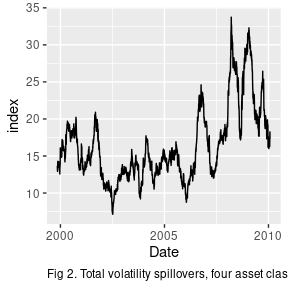This part of the code simply gets all directional spillovers, then we need to plot them.

dy_results <- dynamic.spillover(data=dy2012,
width=200,
p=4) 

### Directional volatility spillovers, FROM four asset classes

In order to get Figure 3 from Diebold and Yilmaz (2012), we proceed as follows:

pp_from <- plotdy(data=dy_results, direction = "from")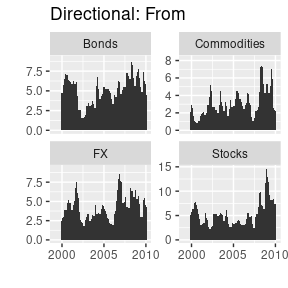pp_from +
labs(caption = "Fig 3. Directional volatility spillovers, FROM four asset classes.")+
theme(plot.caption = element_text(hjust = 0.5))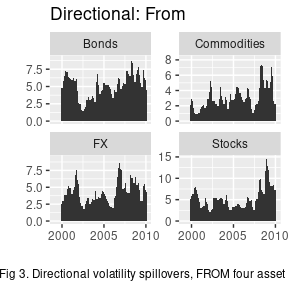### Directional volatility spillovers, TO four asset classes.

Is it difficult to plot directional TO? NO! It is as just easy as:

pp_to <- plotdy(dy_results, direction = "to")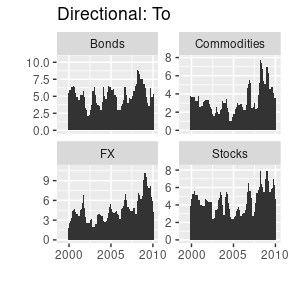# Just for customization
pp_from +
labs(caption = "Fig. 4. Directional volatility spillovers, TO four asset classes.")+
theme(plot.caption = element_text(hjust = 0.5))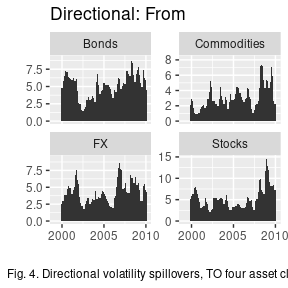If you notice y scale is free, but we can get fix one using facet_wrap(~variables, scales = "fixed")

### Net volatility spillovers, four asset classes.

pp_net <- plotdy(dy_results, direction = "net")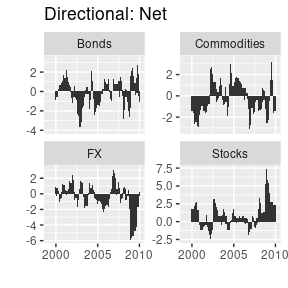pp_net +
labs(caption = "Fig. 5. Net volatility spillovers, four asset classes.")+
theme(plot.caption = element_text(hjust = 0.5))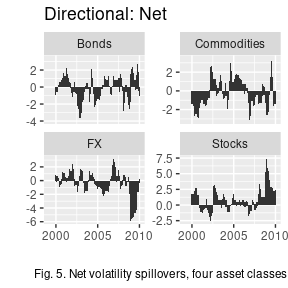### Net pairwise volatility spillovers.

pp_netpairwise <- plotdy(dy_results, direction = "net_pairwise")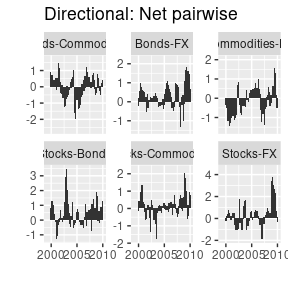pp_netpairwise +
labs(caption = "Fig. 6. Net pairwise volatility spillovers.")+
theme(plot.caption = element_text(hjust = 0.5))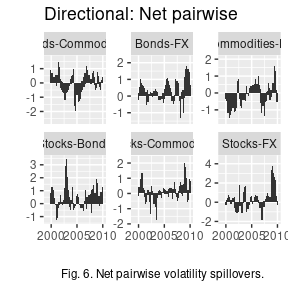### Net pairwise FROM-TO

Finally, we can add a new set of plots:

pp_from_to_pairwise <- plotdy(dy_results, direction = "from_to_pairwise")